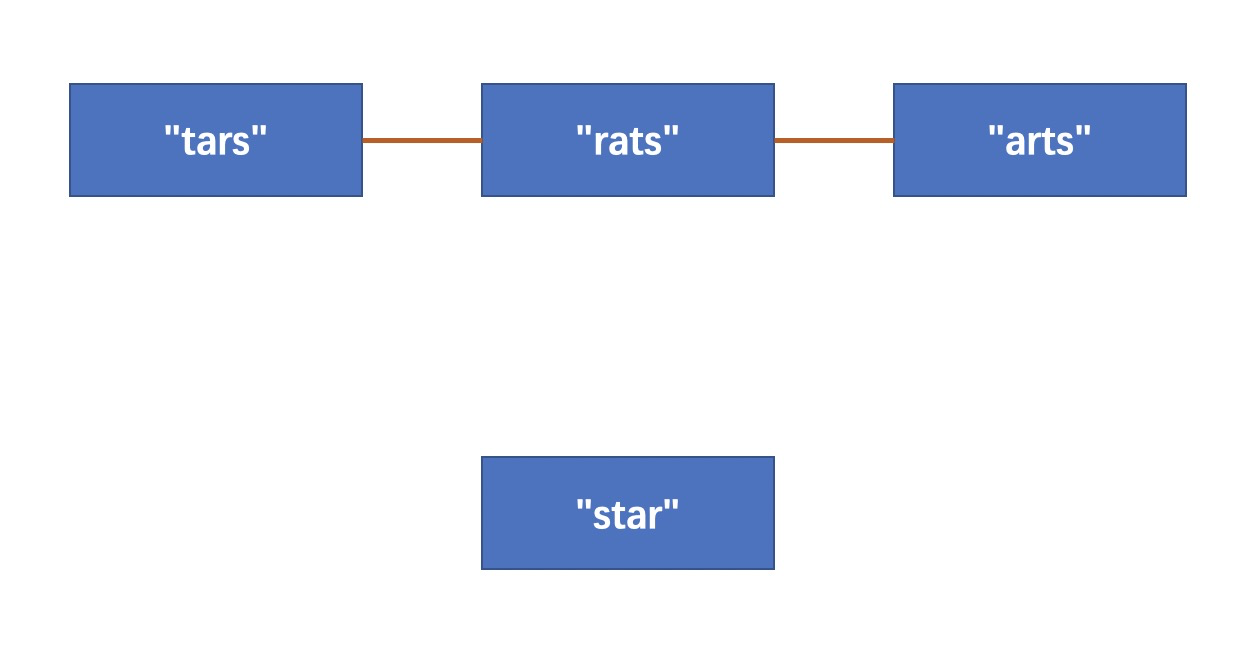# 839. Similar String Groups 相似字符串组

• 作者： 负雪明烛
• id： fuxuemingzhu
• 公众号：负雪明烛
• 本文关键词：LeetCode，力扣，算法，算法题，字符串，并查集，刷题群

@TOC

# # 题目描述

• "tars" 和 "rats" 是相似的 (交换 0 与 2 的位置)； "rats" 和 "arts" 也是相似的。
• 但是 "star" 不与 ["tars"，"rats"，"arts"] 中的任意一个相似，因为无法通过交换 star 中的两个不同位置字母得到三者的任意一个。# # 代码

1. 两重 for 循环，实现对节点之间两两组合，判断两个节点是否相似；
2. 判断相似的方法是：两个字符串的对应位置中只有 0 个或者 2 个不同；
3. 如果两个字符串相似则使用并查集，将此两个节点之间连通上一条边；
4. 统计最终并查集中有多少个不同的连通区域，即为所求。

``````class Solution(object):
def numSimilarGroups(self, strs):
"""
:type strs: List[str]
:rtype: int
"""
N = len(strs)
dsu = DSU(N)
for i in range(N):
for j in range(i + 1, N):
if self.isSimilar(strs[i], strs[j]):
dsu.union(i, j)
return dsu.regions()

def isSimilar(self, str1, str2):
count = 0
for i in range(len(str1)):
if str1[i] != str2[i]:
count += 1
return count == 2 or count == 0

class DSU:
def __init__(self, N):
self.par_ = range(N + 1)
self.regions_ = N

def find(self, x):
if x != self.par_[x]:
self.par_[x] = self.find(self.par_[x])
return self.par_[x]

def union(self, x, y):
px = self.find(x)
py = self.find(y)
if px == py:
return
self.par_[px] = py
self.regions_ -= 1

def regions(self):
return self.regions_
``````

# # 刷题心得

OK，这就是本次题解的全部内容了，如果你觉得我的题解对你有帮助的话，求赞、求关注、求转发、求在看。你的认可就是我前进的最大动力！我们明天再见！

# # 欢迎加入组织# # 日期

2021 年 1 月 31 日 —— 周末加油！努力！